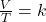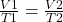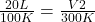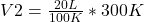Question

The Temperature of 20 liters of helium gas is increased from 100°K to 300°K. If the pressure was kept constant, the new volume will be how many liters? a) 6.7 b) 30 c) 120 e) None of these

1.minhkhang

The new volume will be 60 liters, so the answer is e) None of these

Explanation:

Charles’s Law consists of the relationship between the volume and temperature of a certain amount of ideal gas, which is maintained at a constant pressure, by means of a proportionality constant that is applied directly.

In other words, the Law of Charles establishes that the volume is directly proportional to the temperature of the gas: If the temperature increases, the volume of the gas increases and if instead the temperature of the gas decreases, the volume decreases.

This is mathematically represented by the quotient that exists between volume and temperature, which will have the same value:Assuming that you have a certain volume of gas V1 that is at a temperature T1 at the beginning of the experiment, if you vary the volume of gas to a new value V2, then the temperature will change to T2, and it will be true:In this case:

• V1= 20 L
• T1=100 K
• V2= ?
• T2=300 K

Replacing:Solving:V2= 60 L

The new volume will be 60 liters, so the answer is e) None of these